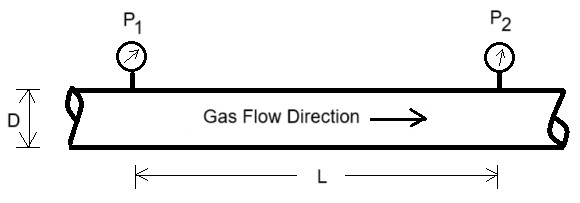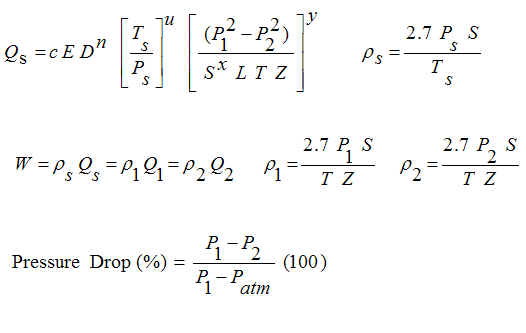Weymouth, Panhandle A and B equations for Compressible Gas Flow

For high pressure gas. Compute Flow, Diameter, Length, Pressures.

Register to enable "Calculate" button.

 Specific Gravity, S: Compressibility, Z: Efficiency Factor, E: Weymouth equation Panhandle A equation Panhandle B equation Temperature, T (F): Air Butane Carbon dioxide Hydrogen Methane Natural Gas Nitrogen Oxygen Propane User enters S Mass Flow, W (lb/s): Compute Flow W known. Compute D Qs known. Compute D W known. Compute L Qs known. Compute L W known. Compute P1 Qs known. Compute P1 W known. Compute P2 Qs known. Compute P2 Std Flow, Qs (std MMcfd): www.LMNOeng.com Diameter, D (inch): Temperature in Fahrenheit, F Temperature in Rankine, R Temperature in Celsius, C Temperature in Kelvin, K Pipe Length, L (mile): Mass Flow in slug/s Mass Flow in lb/s Mass Flow in lb/hr Mass Flow in lb/day Mass Flow in kg/s Mass Flow in kg/hr Mass Flow in kg/day P1 (psig): Vol Flow in ft3/s, cfs Vol Flow in ft3/min, cfm Vol Flow in ft3/hr, cfh Vol Flow in ft3/day, cfd Vol Flow in Thousand cfh, Mcfh Vol Flow in Million cfd, MMcfd Vol Flow in m3/s Vol Flow in m3/min Vol Flow in m3/hr Vol Flow in m3/day P2 (psig): Diameter in inch Diameter in ft Diameter in cm Diameter in m Q1 (actual MMcfd): Length in ft Length in mile Length in m Length in km Q2 (actual MMcfd): Enter P1, P2 as absolute Enter P1, P2 as gage P1-P2 (psi): Pressures in psi Pressures in inch H2O (60F) Pressures in inch Mercury (60F) Pressures in millibars Pressures in N/m2 Pressures in mm Mercury (0C) Pressure Drop (%): Velocity in ft/s; Area in ft2 Velocity in m/s; Area in m2 Upstream Velocity, V1 (ft/s): © 2015 LMNO Engineering, Downstream Velocity, V2 (ft/s): Research, and Software, Ltd. Pipe Area, A (ft2):Units: acfd=actual cfd, acfh=actual cfh, acfm=actual cfm, cfd=cubic foot per day, cfh=cubic foot per hour, cfm=cubic foot per minute, cfs=cubic foot per second, cm=centimeter, hr=hour, kg=kilogram, km=kilometer, lb=pound, m=meter, mm=millimeter, Mcfh=thousand cfh, MMcfd=million cfd, N/m2=Newton per square meter (same as Pascal), s=second, psi=pound per square inch, psia=psi (absolute), psig=psi (gage), scfd=std cfd, scfh=std cfh, scfm=std cfm, std=standard conditions (60F, 14.7 psia).

Introduction
The Weymouth, Panhandle A, and Panhandle B equations were developed to simulate compressible gas flow in long pipelines. The Weymouth is the oldest and most common of the three. It was developed in 1912. The Panhandle A was developed in the 1940s and Panhandle B in 1956 (GPSA, 1998). The equations were developed from the fundamental energy equation for compressible flow, but each has a special representation of the friction factor to allow the equations to be solved analytically. The Weymouth equation is the most common of the three - probably because it has been around the longest. The equations were developed for turbulent flow in long pipelines. For low flows, low pressures, or short pipes, they may not be applicable.

If the pressure drop in a pipeline is less than 40% of P1, then our Darcy-Weisbach incompressible flow calculation may be more accurate than the Weymouth or Panhandles for a short pipe or low flow. The Darcy-Weisbach incompressible method is valid for any flow rate, diameter, and pipe length, but does not account for gas compressibility. Crane (1988) states that if the pressure drop is less than 10% of P1 and you use an incompressible model, then the gas density should be based on either the upstream or the downstream conditions. If the pressure drop is between 10% and 40%, then the density used in an incompressible flow method should be based on the average of the upstream and downstream conditions. If the pressure drop exceeds 40% of P1, then use a compressible model, like the Weymouth, Panhandle A, or Panhandle B. You can always run all models and compare the results. The calculation is only valid for subsonic velocities. It does not check if velocities are sonic or supersonic.

Equations
The equations are shown below. The Weymouth, Panhandle A, and Panhandle B equations (GPSA, 1998; Crane, 1988) are the equation beginning with Qs=...  with the constants c, n, u, x, and y defined below. All of the equations shown below use the English units indicated in the Variables section. Of course, our calculation uses a variety of units with all of the unit conversions handled internally by the program.Variables:
The units refer to the units that must be used in the equations shown above. However, a variety of units may be used in our calculation.
A = Pipeline cross-sectional area.
c = Constant. Weymouth: c=18.0625, Panhandle A: c=18.16125, Panhandle B: c=30.7083
D = Pipe inside diameter [inch].
E = Efficiency factor. Typically 0.85 to 1.0. The lower the number, the rougher (or older) the pipe. Typical value is 0.92.
L = Pipeline length between locations 1 and 2 [mile].
n = Constant. Weymouth: n=2.6667, Panhandle A: n=2.6182, Panhandle B: n=2.53
P = Absolute pressure in pipeline [psia, i.e. pounds per square inch absolute].
Q = Volumetric flowrate [cfh, i.e. cubic foot per hour]
S = Specific gravity of gas in pipeline, relative to air. That is, the ratio of the gas's molecular weight to the molecular weight of air [unit-less].
T = Absolute temperature [Rankine]. Note: oR=oF+459.67
u = Constant. Weymouth: u=1.0, Panhandle A: u=1.0788, Panhandle B: u=1.02
V = Velocity of gas = Q/A.
W = Mass flowrate [lb/hr, i.e. pounds per hour].
x = Constant. Weymouth: x=1.0, Panhandle A: x=0.853, Panhandle B: x=0.961
y = Constant. Weymouth: x=0.5, Panhandle A: x=0.5392, Panhandle B: x=0.51
Z = Gas compressibility. Value typically 1.0 at standard conditions. Typically decreases as pressure increases. Can be as low as 0.4 or so. Exact computation depends on make-up of the gas, gas critical pressure and temperature, and actual temperature and pressure.
ρ = Greek letter rho. Density [lb/ft3, i.e. pounds per cubic foot]. Note that the equation for ρs uses a value of 1.0 for the compressibility.

Subscripts:
1 = Upstream conditions.
2 = Downstream conditions.
atm = Atmospheric conditions (14.73 psia).
s = Standard conditions (520 R, 14.73 psia)

Error Messages given by calculation
"Need D>0", "Need L>0", "Need S>0", "Need Qs>0", "Need W>0", "Need E,Z > 0".  These are initial checks. Diameter, length, specific gravity, and flows must be positive numbers.

"Need T>0 K", "Need P1>0 absolute", "Need P2>0 absolute", "Need P1>P2".  Temperature must be greater than absolute zero. Pressures must be greater than 0 absolute. And, pressure difference must be positive. Since you may have entered temperature in other units or pressure as gage, the calculation does some initial computations to determine the validity of the input.

"P1 too small".  This message appears if P2 is computed as less than 0 absolute.

References
Crane Co. 1988. Flow of Fluids through Valves, Fittings, and Pipe. Technical Paper 40 (TP-40). http://www.craneco.com

GPSA (Gas Processors Suppliers Association). Engineering Data Book. 11ed. 1998. https://gpsa.gpaglobal.org

LMNO Engineering, Research, and Software, Ltd.
7860 Angel Ridge Rd.   Athens, Ohio 45701  USA   Phone: (740) 592-1890
LMNO@LMNOeng.com    http://www.LMNOeng.com

To: